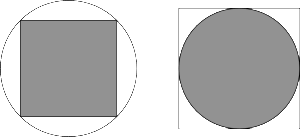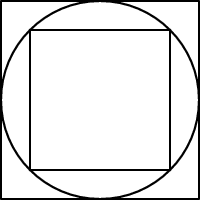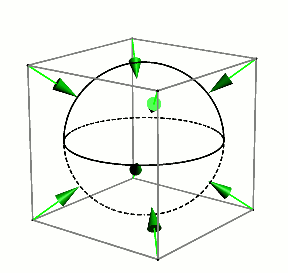# In what flipping dimension is a square peg in a round hole just as good as a round peg in a square hole?

In what flipping dimension is a square peg in a round hole just as good as a round peg in a square hole?

Let’s start at the beginning.

My Plus magazine puzzle from March asks “Which gives a tighter fit: a square peg in a round hole or a round peg in a square hole?” By “tighter” we mean that a higher proportion of the hole is occupied by the peg.Note that everything is scalable when considering fit: think of shrinking or expanding the images above with a photocopier; it’s only the ratios of areas that are important, not the specific measurements.

This question has been asked and answered many times before, and in generalised form too (more on that below).

One could also ask which is a better fit on the internet, by comparing Google hits for “round peg in a square hole” versus “square peg in a round hole”. The latter has been gaining popularity for over a century, at least since Edward Bulwer Lytton wrote

You … insist upon forcing a square peg into a round hole, because in a round hole you, being a round peg, feel tight and comfortable. Now I call that irrational.

Have those who use this phrase considered the alternative above, and done the maths? Probably not.

Ponder this related puzzle.What portion of the large square does the small square cover?

The answer to that one is “one half” — just imagine that the inner square is rotated $45^{\circ}$ and it becomes pretty clear, without any computation at all. This observation allows us to answer our question too.

Assume that the larger square in the triple nested image above has width $2$, and hence area $4$. Upon reflection—and rotation, as just suggested—the smaller inside square must have area $2$ (an application of Pythagoras also confirms this). The circle has area $\pi$ of course.

So the square peg in a round hole fit is $\frac{2}{\pi}$, and the round peg in a square hole fit is $\frac{\pi}{4}$.

Since $\pi > 3$ it follows that $\pi^2 > 8$, and hence $\pi > \frac{8}{\pi}$. Dividing by $4$ yields $\frac{\pi}{4} > \frac{2}{\pi}$.

Hence, a round peg in a square hole is a better fit than a square peg in a round hole!As decimal approximations, we are comparing $0.7854$ with $0.6366$: a round peg fills up about 78.54% of a square hole, whereas a square peg only fills up about 63.66% of a round hole.

Maybe that’s why we sometimes see round tins in rectangular boxes, but square bottles such as those pictured on the right are rarely packed in cylindrical boxes, since the latter is a less economical use of space.

If we’re going to move up a little in dimension, from $2$, let’s go all the way to $3$, and ask:

Is a sphere in a cube also a better fit than a cube in a sphere? Can you do the appropriate computations in this case? Does it help to consider the 3D analogue of the nested image above, this time of a cube in a sphere in a cube, and then rotate the inner cube?

It’s fun to envision a sphere starting inside a cube and then expanding until it perfectly encloses the cube.It turns out that we are comparing $\frac{\pi}{6}$ (52.36% filled) and $\frac{2}{\pi \sqrt{3}}$ ( 36.76% filled)

What about in dimension $4$? That’s a little harder for most of us to visualise, unless you have the 4D instincts of somebody like geometer Tom Banchoff! Is a $4$-ball a better fit in a $4$-cube than a $4$-cube in a $4$-ball?

Here, it turns out that we are comparing $\frac{\pi^2}{32}$ (30.84% filled) and $\frac{2}{\pi^2}$ ( 20.26% filled)

Notice that we are getting poorer fits in both cases as the dimension goes up. It gets worse (before it gets weird).